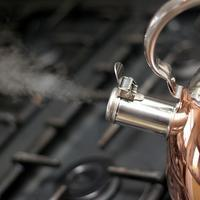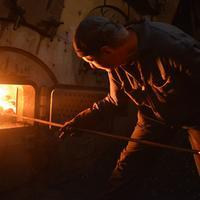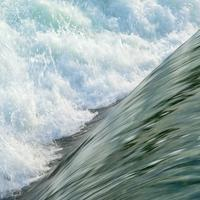# O: Engineering

## What to expect

Engineering is an applied science, which uses an understanding of systems to design tools and engines that can assist mankind.

The IB Physics course contains two key sections at Standard Level:

At Advanced Higher Level, you will study fluids and fluid dynamics and damped and driven oscillations.## Key questions

#### What is torque?

Torque, also known as the moment of a force, is defined as the product of a force and the perpendicular distance from the line of action to the pivot. Find out more.

#### What is meant by rotational dynamics?

Using a similar process of rearranging and substitution to the suvat equations of linear motion, we can derive equations for rotational motion. Find out more.

#### What are the laws of thermodynamics in simple terms?

The first law of thermodynamics states that the amount of heat that enters a closed gas container is equal to the sum of the increase in internal energy and the work done by the gas on the surroundings. Find out more.

The second law of thermodynamics can be expressed in three ways.

1. Clausius: Heat cannot pass from a colder to a warmer body without work being done.
2. Kelvin: It is impossible to convert all heat into mechanical work during a cyclic process with 100% efficiency.
3. Entropy: In any isolated system, entropy will either remain the same or increase.

Find out more.

#### What is Bernoulli's principle?

Bernoulli's principle states that an increase in the speed of a fluid occurs simultaneously with a decrease in pressure or a decrease in the fluid's potential energy. Find out more.

#### What is the difference between turbulent and laminar flow?

In laminar flow, particles in the fluid follow smooth paths in layers with no mixing. Motion is orderly with particles close to the walls of a pipe moving in straight lines parallel to the walls. Laminar flow occurs at low velocities in fluids of high viscosity, below a Reynolds number threshold at which the flow becomes turbulent. Stokes' law applies and drag forces are low. In turbulent flow, fluid particles experience changes in pressure and flow velocity due to excessive kinetic energy in a fluid of low viscosity. Vortices and eddy currents develop causing mixing of the layers of fluid. The Reynolds number exceeds the threshold for laminar flow and Stokes' law does not apply. Find out more.

#### How do you calculate Q factor?

The Q (quality) factor for oscillations is a dimensionless quantity that indicates the extent of damping. It is the ratio of the energy stored at the beginning of a cycle to the energy lost in the system as a result of that same cycle multiplied by 2π. Find out more.

•### Torque and moment of inertia

Torque, also known as the moment of a force, is defined as the product of a force and the perpendicular distance from the line of action to the pivot.

•### Equilibrium and angular acceleration

A body is in rotational equilibrium if the sum of the anticlockwise torques is equal to the sum of the clockwise torques about a pivot.

•### Rotational dynamics

An object experiences angular acceleration if it is not in rotational equilibrium. The equations for linear motion have rotational equivalents.

•### Angular momentum and rotational kinetic energy

The rotational equivalent of linear momentum is angular momentum. It is defined as the product of moment of inertia and angular velocity. We can also calculate rotational kinetic energy.

•### First law

Thermodynamics is the study of the movement of heat and its relationship with work.

•### Processes

We can use diagrams to show the processes that take place in a container of gas. A graph of pressure vs volume enables us to show all possible types of process.

•### Second law

A heat engine converts thermal energy into mechanical work. The second law of thermodynamics can be expressed in three ways.

•### Cyclic processes

A cyclic process is a series of transformations that take the gas back to its original state. These form a closed loop on a pV diagram.

•### AHL Static fluids

Don't be fooled into thinking that only solids are 'heavy' and that we can approximate everything as a volume-less particle. Fluids, such as liquids, have a density, exert an upward buoyancy on anything that should displace them, have increasing pressure w

•### AHL Fluid dynamics

Fluid dynamics is the study of moving fluids. As with moving bodies, mass and energy are conserved leading to equations that can be used to determine unknown quantities. An understanding of fluid properties enables us to calculate drag forces and the categ

•### AHL Damped and driven oscillations

In the simple harmonic motion topic we considered natural oscillations of pendula and masses on springs. But so far we have ignored the effect of the fluid in which the oscillations are taking place and therefore the effects of damping. And what if these o